Processing ......FreeComputerBooks.com Links to Free Computer, Mathematics, Technical Books all over the World

Complex Integration and Cauchy's Theorem
Top Free Web Programming Books 🌠 - 100% Free or Open Source!
• Title Complex Integration and Cauchy's Theorem
• Author(s) G.N. Watson
• Publisher: Dover Publications (April 19, 2012); Cambridge University Press (1914)
• Hardcover/Paperback 96 pages
• eBook PDF (100 pages, 3.2 MB), ePub, Kindle, Daisy, DjVu, etc.
• Language: English
• ISBN-10: 0486488144
• ISBN-13: 978-0486488141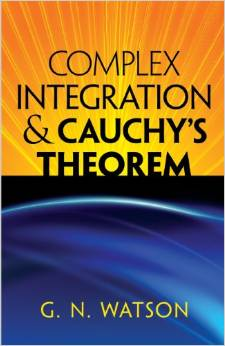Book Description

This brief monograph by one of the great mathematicians of the early twentieth century offers a single-volume compilation of propositions employed in proofs of Cauchy's theorem. Developing an arithmetical basis that avoids geometrical intuitions, Watson also provides a brief account of the various applications of the theorem to the evaluation of definite integrals.

Author G. N. Watson begins by reviewing various propositions of Poincare's Analysis Situs, upon which proof of the theorem's most general form depends. Subsequent chapters examine the calculus of residues, calculus optimization, the evaluation of definite integrals, and expansions in series. A historical summary concludes the text, which is supplemented by numerous challenging exercises.

• G.N. Watson was an English mathematician, who applied complex analysis to the theory of special functions.
Reviews, Ratings, and Recommendations: Related Book Categories: Read and Download Links:Similar Books:
•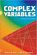Complex Variables: Second Edition (Robert B. Ash, et al)

This text on complex variables is geared toward who have taken an introductory course in real analysis. Offering a concise treatment that provides careful and complete explanations as well as numerous problems and solutions.

•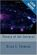Theory of the Integral (Brian S. Thomson)

This text is intended as a treatise for a rigorous course introducing the elements of integration theory on the real line. All of the important features of the Riemann integral, the Lebesgue integral, and the Henstock-Kurzweil integral are covered.

•Calculus (Gilbert Strang)

This highly regarded calculus textbook is ideal as for self-study. Examples of apps of calculus to physics, engineering and economics are included, as well as many practice questions and illustrative diagrams to assist the reader's grasp of the material.

•Measure, Integration and Real Analysis (Sheldon Axler)

This textbook welcomes students into the fundamental theory of measure, integration, and real analysis. Focusing on an accessible approach, it lays the foundations for further study by promoting a deep understanding of key results.

•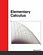Elementary Calculus (Michael Corral)

This textbook covers calculus of a single variable, is designed for students who have completed courses in high-school algebra, geometry, and trigonometry. Though designed for college students, it could also be used in high schools.

•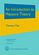An Introduction to Measure Theory (Terrence Tao)

This is a graduate text introducing the fundamentals of measure theory and integration theory, which is the foundation of modern real analysis, intended to cover a quarter or semester's worth of material for a first graduate course in real analysis.

•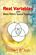Real Variables with Basic Metric Space Topology (Robert B. Ash)

Designed for a first course in real variables, this text presents the fundamentals for more advanced mathematical work, particularly in the areas of complex variables, measure theory, differential equations, functional analysis, and probability.

•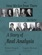How We Got from There to Here: A Story of Real Analysis

This book is an introductory real analysis textbook, presented through the lens of history. The definitions and techniques are motivated by the actual difficulties encountered by the intuitive approach and are presented in their historical context.

Book Categories
 :All CategoriesTop Free BooksRecent BooksMiscellaneous BooksComputer EngineeringComputer LanguagesComputer ScienceData Science/DatabasesJava and Java EE (J2EE)Linux and UnixMathematicsMicrosoft and .NETMobile ComputingNetworking and CommunicationsSoftware EngineeringSpecial TopicsWeb Programming
Other Categories